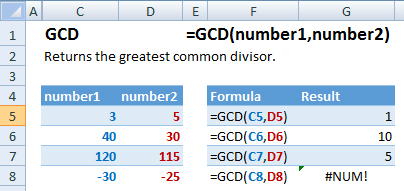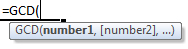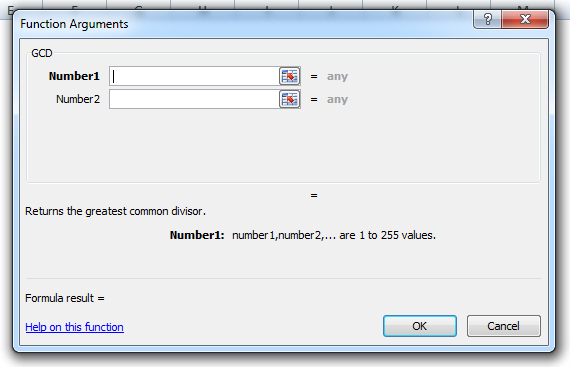# GCD Function Examples – Excel, VBA, & Google Sheets

This Excel Tutorial demonstrates how to use the Excel GCD Function in Excel to calculate the greatest common divisor, with formula examples.

## GCD Function Description:

The GCD Function Calculates the greatest common divisor between two or more numbers. The greatest common divisor is the largest integer that will divide into all of the numbers.

## Formula Examples:Example Formula Result
1 =GCD(C5,D5) 1
2 =GCD(C6,D6) 10
3 =GCD(C7,D7) 5
4 =GCD(C8,D8) #NUM!

## Syntax and Arguments:

The Syntax for the GCD Formula is:

``=GCD(number1,number2)``

### Function Arguments ( Inputs ):

number1 – Two or more numbers, separated by commas, or an array of numbers.

Use the GCD Function to calculate the greatest common divisor for a number. Simply enter any number directly into the formula, or reference a cell containing a number.

## GCD Examples in VBAYou can also use the GCD function in VBA. Type:
`Application.Worksheetfunction.Gcd(number1,number2)`
For the function arguments (number1, etc.), you can either enter them directly into the function, or define variables to use instead.

`=AND(`